Home > CCA2 > Chapter 8 > Lesson 8.3.3 > Problem8-171

8-171.
1. Generally, when you are asked to factor, it is understood that you are only to use integers in your factors. If you are allowed to use irrational or complex numbers, any quadratic can be factored.

2. By setting the polynomial equal to zero and solving the quadratic equation, you can work backwards to “force factor” any quadratic. Use the solutions of the corresponding quadratic equation to write each of the following expressions as a product of two linear factors. Homework Help ✎

1. x2 − 10

2. x2 − 3x − 7

3. x2 + 4

4. x2 − 2x + 2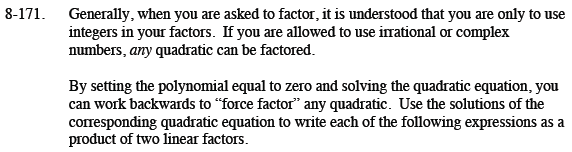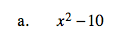x2 − 10 = 0

x2 = 10

$x= \pm \sqrt{10}$

$(x + \sqrt{10})(x - \sqrt{10})$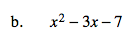Use the Quadratic Formula, then use those solutions as factors.

$\left(x - \frac{3 + \sqrt{37}}{2}\right)\left(x- \frac{3 - \sqrt{37}}{2}\right)$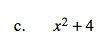Follow the steps outlined in part (a).

$(x + 2i)(x - 2i)$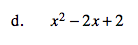See part (b).